# Design and Simulation of Sol-Gel-Based Electro Optic Modulator

## Introduction

Because of its large bandwidth, ease of process, and relatively low cost, electro-optic (EO) modulators based on sol-gel are an attractive replacement to LiNbO3-based EO modulators. Modeling and simulation plays a critical role in the design & optimization of such devices. This application note will show how such a complex device can be decomposed into a few basic functional blocks, designed and optimized individually, and finally verified by using RSoft BeamPROP.

## Overall Simulation Strategy

Shown in Figure 1 is the example structure to be used .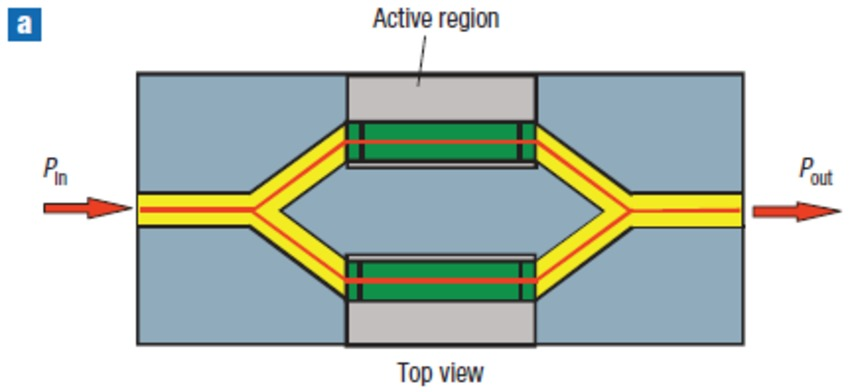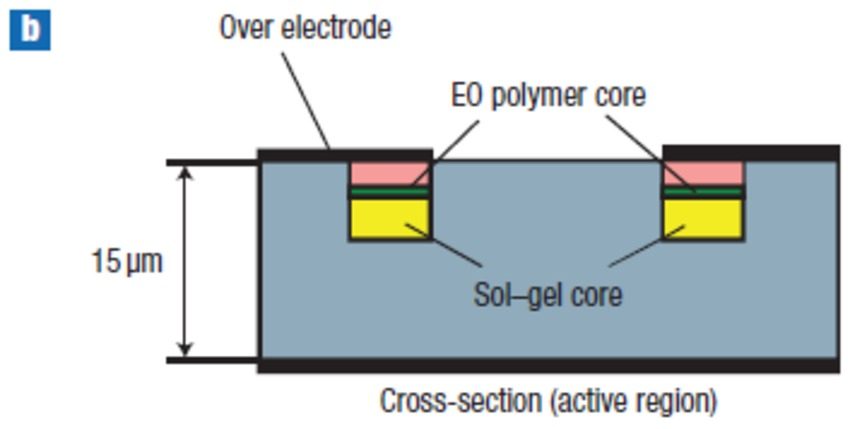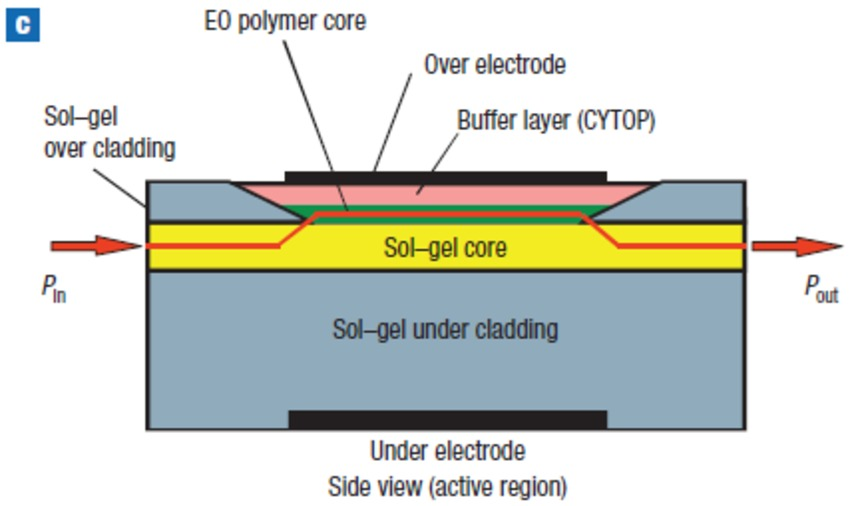Figure 1. The device structure. a, top view of the device; b, cross-section of the active region; c, side view of the device.

As observed, this complex structure consists of a few basic functional blocks:

• Passive and active waveguides,
• Spot-size converter between two kinds of waveguide
• Y-branches for power splitter and combiner
• E-O phase shifter

Each building block is critical and should be designed and optimized properly to ensure the overall device performance.

## Design of Functional Elements

As proposed earlier, the E-O modulator will be decomposed into a number of basic functional elements to be designed individually. The material properties will be the first step for every element.

## Materials

Based on the references [2,3], sol-gel materials consist of a precursor and an index modifier. Therefore, the material model of the sol-gel can be treated as its precursor with a small index change, similar to the way that doped silica for optical fiber would be. The benefit of this approach is that same material dispersion property, which is essential for some applications such as traveling wave modulation, can be used for both the precursor and the modified materials.

For this example, unfortunately, there is no material dispersion data reported in  or readily found in literature. We will use the data reported in ; the refractive indices at 1550nm are 1.500, 1.487, 1.643, and 1.328 for sol-gel core, sol-gel cladding, polymer, and top buffer layer.

In addition, the electrical properties of the material, namely the dielectric constant, is also essential to model the electric behavior of the E-O device. The dielectric constant of the sol-gel core and cladding is 3.0, based on reference . The dielectric constant of buffer material CYTOP is ~2.0 based on the datasheet from Bellex . The polymer used in ref  is AJLS102 polycarbonate, and its dielectric constant is ~2.9 .

This modulator is based on the E-O effect, hence the E-O coefficient of the polymer has to be known. Based on , the E-O coefficients are R33=170pm/V and R13= R33/3. Please note that the “crystal” orientation of the polymer (x’,y’,z’) corresponds to RSoft coordinates (x,z,y), therefore, the E-O coefficients defined in RSoft Material Editor are Rx=Rz=0, and Ry= R13 or R33 for TE or TM polarizations, as shown in Figure 2.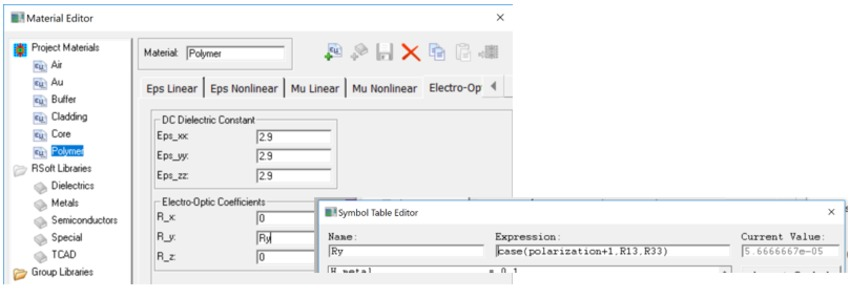Figure 2. Definition of material properties in the RSoft Material Editor.

## Passive Waveguide

As indicated in the Figure 1, the passive waveguide is a buried channel waveguide with sol-gel core buried in lower index sol-gel cladding. Based on reference , the core height is 3.5µm and the waveguide width was not mentioned; we will use 4µm in the design example.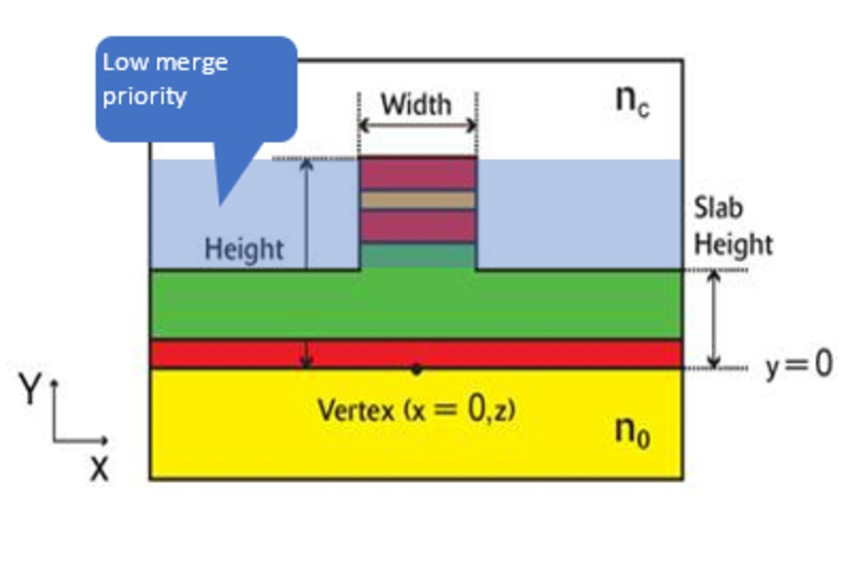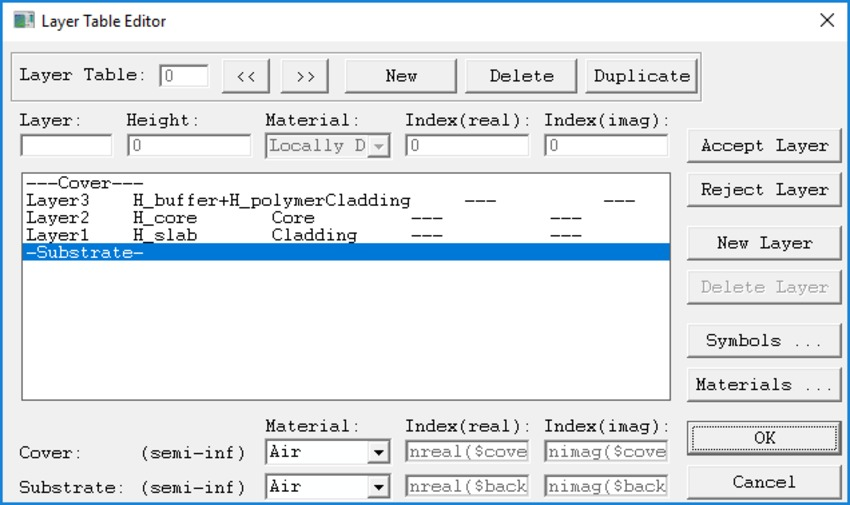Figure 3. Constructing waveguide by multilayer and a “filling” channel.

For ease and consistency with the active waveguide, we define the passive waveguide with the built-in “multilayer” waveguide type as shown in Figure 3. Because the default multilayer waveguide assumes that the etched rib/ridge is uncoated, we need to add a channel waveguide, with lower merge priority to fill the etched gap.

The mode can be calculated by RSoft’s built-in BPM mode solver or FemSIM. Shown in Figure 4 are the TE and TM modes calculated by the BPM mode solver. It is observed that they are linearly polarized, hence semi-vector BPM calculation is sufficient and will be used in the future.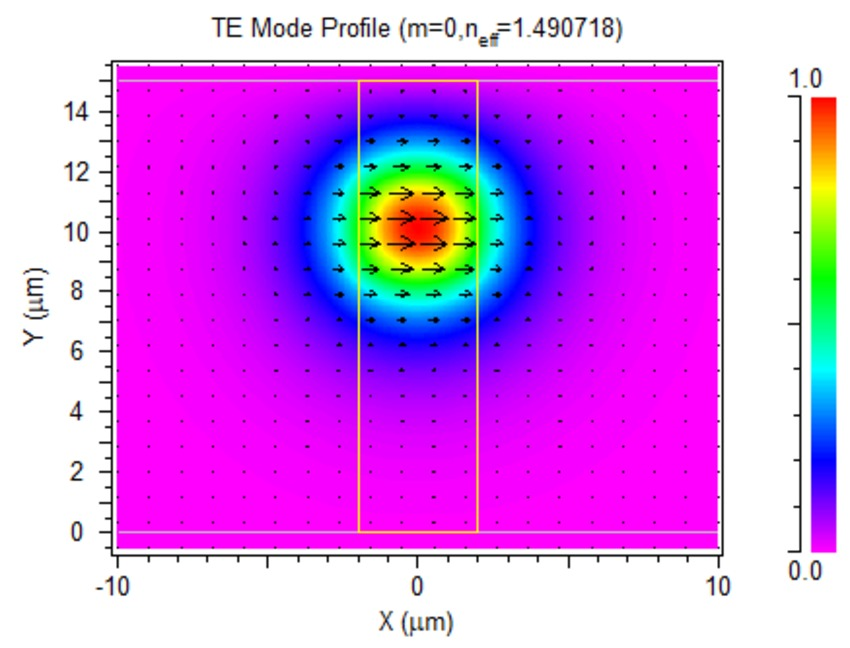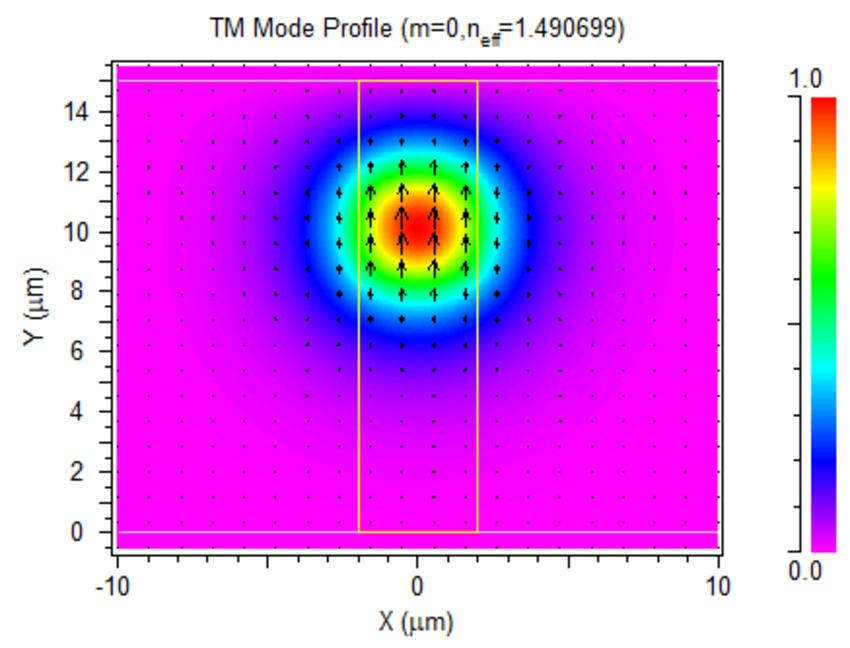Figure 4. Calculated modes supported by the passive waveguide.

The function of the passive waveguide is to provide better coupling with optical fiber. Therefore, the coupling efficiency with a standard optical fiber is one of the design aspects, and it can be easily calculated in by overlapping the calculated mode with a fiber mode. Shown in Figure 5 is the MOST Scan results for coupling efficiency, with the standard Corning SMF-28 fiber as a function of the waveguide width.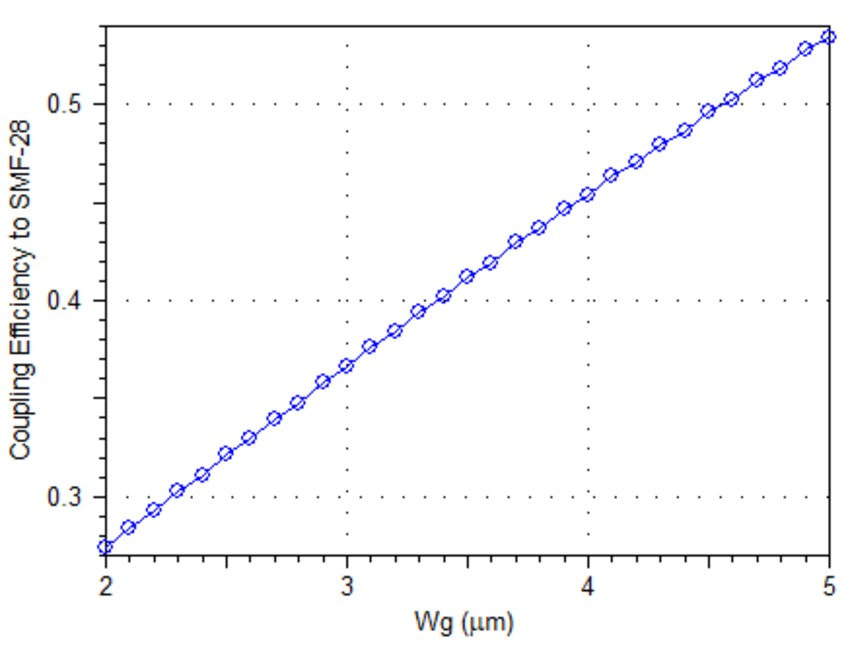Figure 5. Coupling efficiency with Corning SMF-28 fiber.

## Active Waveguide

As shown in Figure 1, the active waveguide is slightly different from the passive waveguide, with two extra layers, an active polymer layer and a buffer layer. Hence, we can define another layer table (Layer 1) for the active waveguide as shown in Figure 6.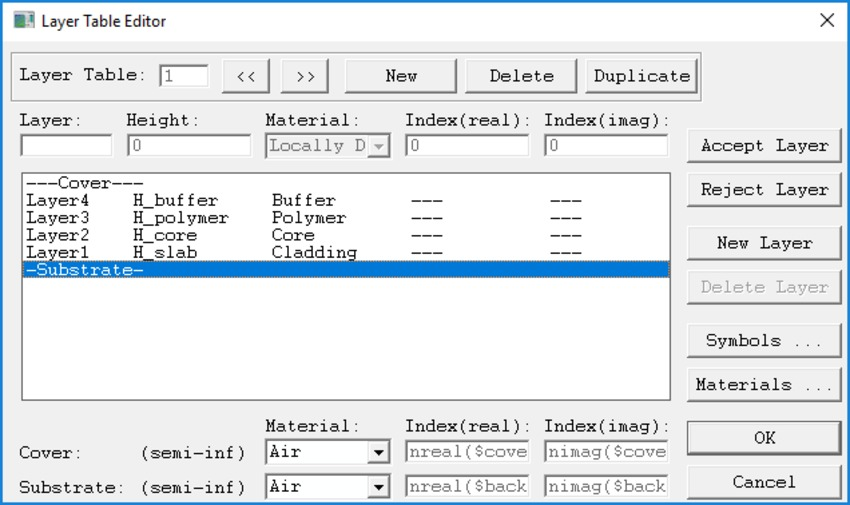Figure 6. Layer table for active waveguide.

Because of the high index of the polymer layer, combined with the existing passive waveguide, the active waveguide is a multi-mode waveguide. FemSIM calculations indicate there are eight (8) guided modes at λ=1.55µm, as shown in Figure 7; six (6) confined in the active region and two (2) in the passive region.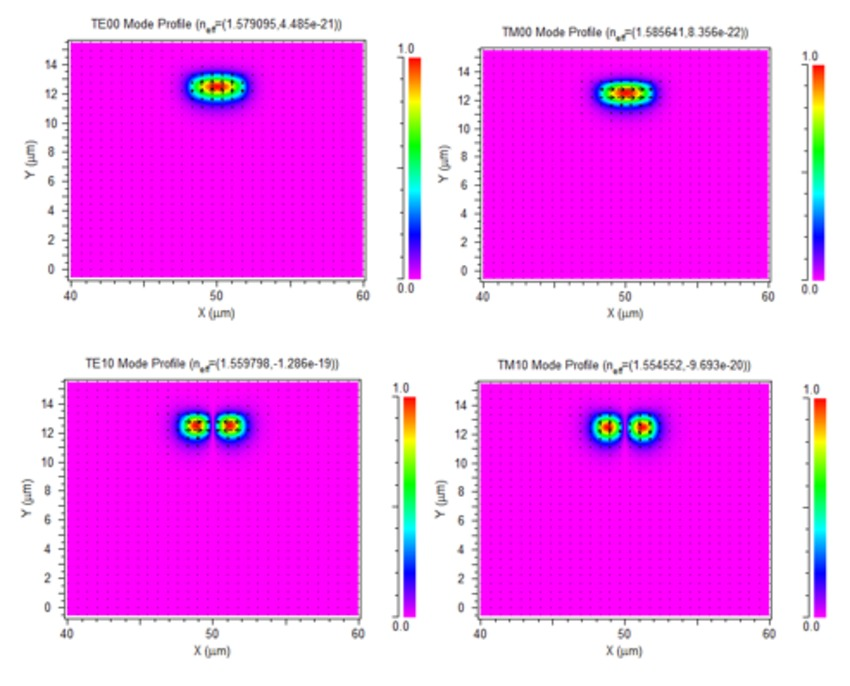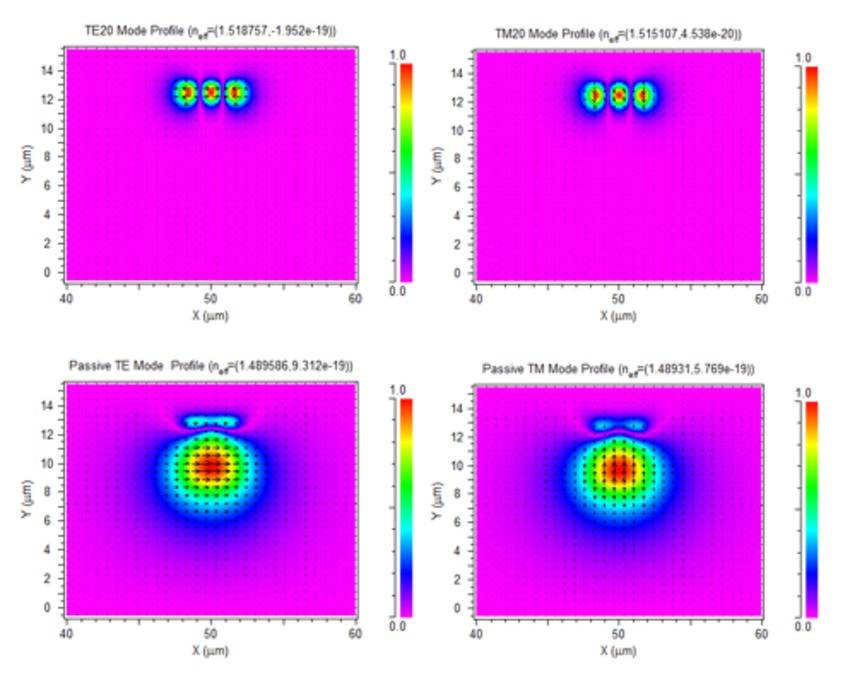Figure 7. Modes guided by the active waveguide.

One of the important characteristics of the active waveguide is the confinement factor (CF), which measures how effectively the active material is modulated electronically. The CF can be easily obtained by creating a pathway in active region and monitoring the partial power. Because the active layer is lumped in the multilayer waveguide, we need to create an inactive channel to cover the active layer and use it as pathway.

The calculated CFs for above eight modes are: 0.8866 (TE00), 0.8734(TM00), 0.8493(TE10), 0.8334(TM10), 0.7483(TE20), 0.7329 (TM20), 0.0362(passive TE), and 0.0253 (passive TM), respectively.  Please note that the CF is higher than the 80% mentioned in , because the waveguide width as well as some material data were not reported in the reference.

## Spot-size Converter

As observed, the modal profile of the fundamental mode of the active waveguide is very different from that of the passive waveguide. Direct butt-to-butt coupling will be very lossy and a spot-size converter (SSC), as shown in Figure 1, is needed to reduce the insertion loss. Because of its slowly varying adiabatic coupling, BeamPROP is an excellent tool to model the light transition.

As shown in Figure 8, the SSC is an overlapping region of the passive waveguide with the active waveguide. In RSoft CAD, the SSC can be easily realized by (1) setting higher merger priority to the passive waveguide so that it can overwrite the active waveguide and (2) Tapering the height of the passive waveguide to create a wedge. Then, we can obtain the desired index profile as shown in Figure 8 (c).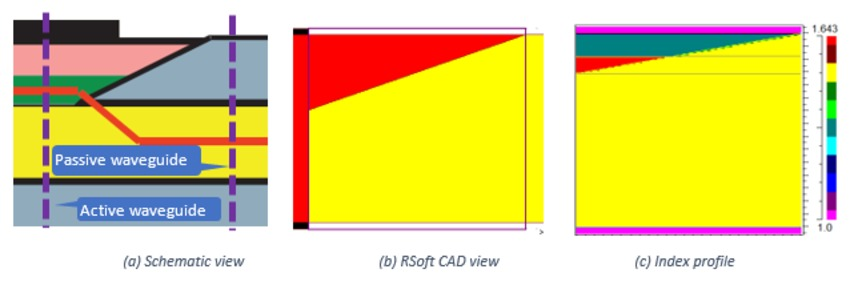Figure 8. Construction of SSC.

The input to the SSC is the computed mode from the passive waveguide designed earlier, as shown in Figure 9 (a). The output is the overlap with the pre-calculated fundamental mode of the active waveguide, either the TE00 or TM00 mode depending on the polarization.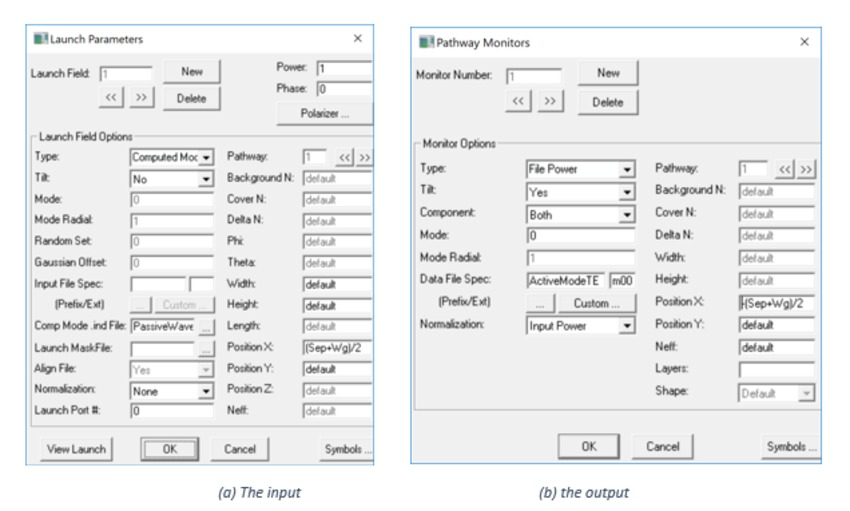Figure 9. Input and output settings for the SSC.

For a 5mm long SSC, the simulation shows it has nearly 100% conversion efficiency, for both TE and TM modes, as shown in Figure 10.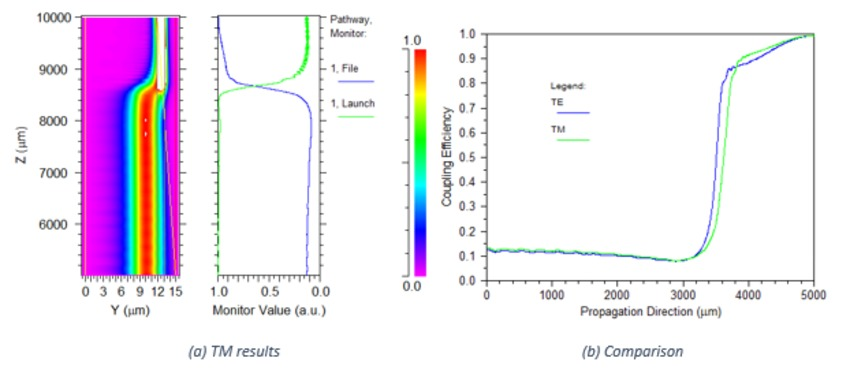Figure 10. BeamPROP simulation of the SSC.

For the SSC, there is always a trade-off between device length and coupling efficiency. To get a general idea of the impact, shown below in Figure 11 is the MOST scan curve over device length. As expected, shorter devices save space, but suffer from lower efficiency.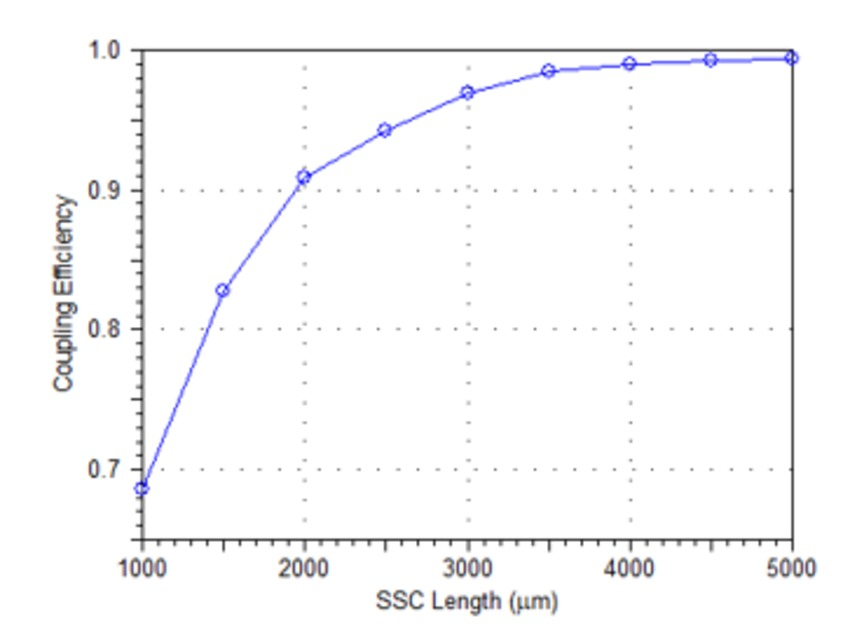Figure 11. SSC conversion efficiency as a function of device length.

## Y-branch

The Y-branch is the simplest 1x2 power splitter or combiner, which consists of two S-bends. The simulation is very straightforward with the computed mode as input and monitors for launch power in both branches. Because of symmetry, power in both branches are the same as shown in Figure 12(a).

There are only two design parameters; device length and separation. It is obvious that smaller separation and longer devices give lower insertion loss. However, in reality, the separation cannot be too small because of optical & electrical crosstalk. The separation should be fixed based on the crosstalk tolerance, then minimum device length to meet the insertion loss can be determined. To do so, we can use MOST to scan both design parameters, and obtain the design map as shown in Figure 12(b).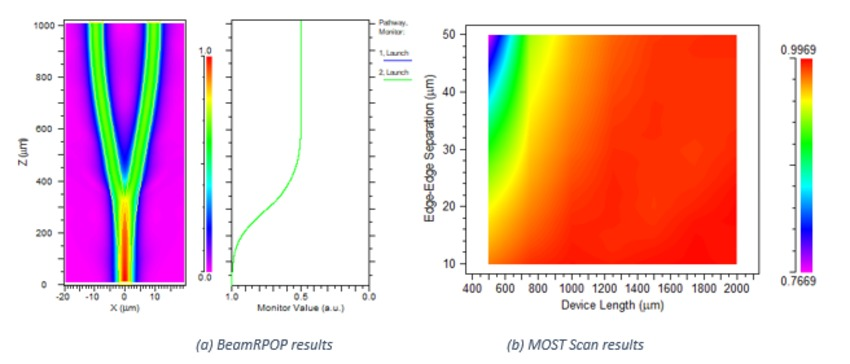Figure 12. Simulation results of the Y-branch power splitter.

The Y-splitter can also be used reversely as a combiner. To test the on-off function, we launched two inputs from both branches with a phase difference. Shown in Figure 13(a) is the simulation result when the phase difference is 180o ; note that the Y-branch behaves exactly as it would at the off-state.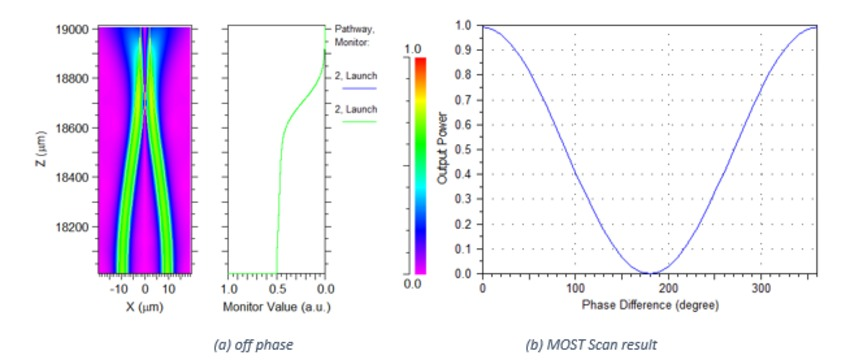Figure 13. Simulation results of the Y-branch combiner.

MOST scan results over the phase difference shows how the combiner evolves between on-state and off-state, as shown in Figure 13(b).

## Electro-Optic Phase Shifter

The phase shifter is the most critical and also the most complex element. It involves both optics and electronics. BeamPROP remains the optical solver and the Multi-Physics Electro-Optical Utility will be used for the electronic solver.

A push-pull configuration is used for the modulator as shown in Figure 14. Three electrodes are biased by -V, 0V, and +V, respectively. Shown on the left are the Multi-Physics Utility calculation results.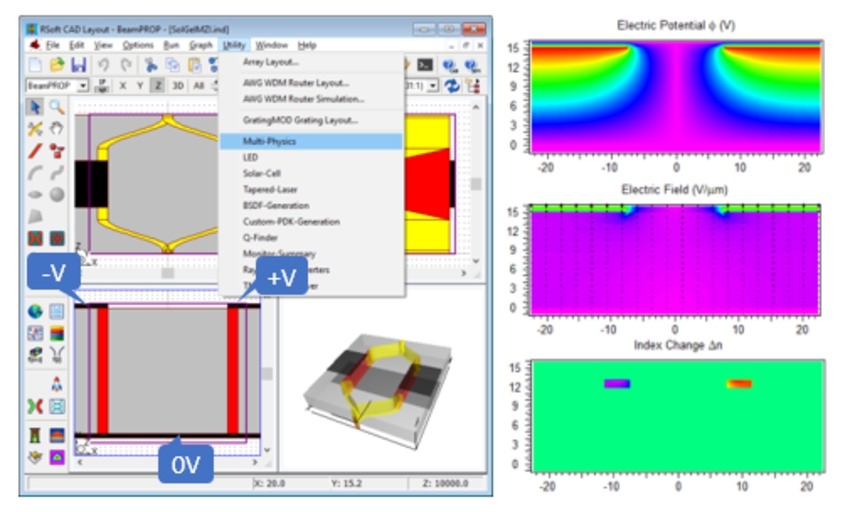Figure 14. Multi-Physics Utility settings and calculation results.

Please note that the computation domain for electronic solver has to be large enough to cover all electrodes. The optical solver, however, can be performed at much smaller scale around the optical waveguide.

The most important characteristics of a phase shifter is the half-wave length Lπ, which is defined as the device length to accumulate 180o phase shift under a given bias.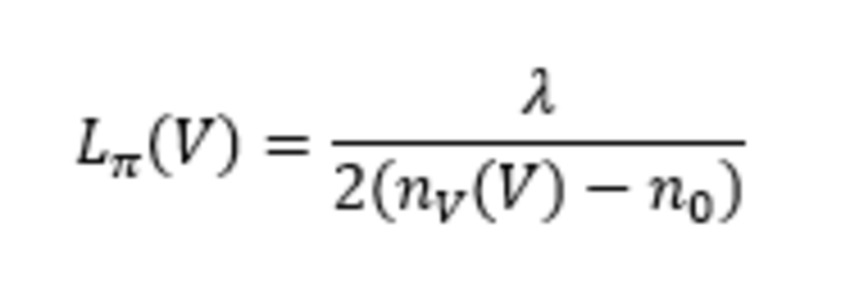Where nv(V), and n0 are the effective indices of the mode with and without bias. Therefore, we can define a MOST metric as shown in Figure 15.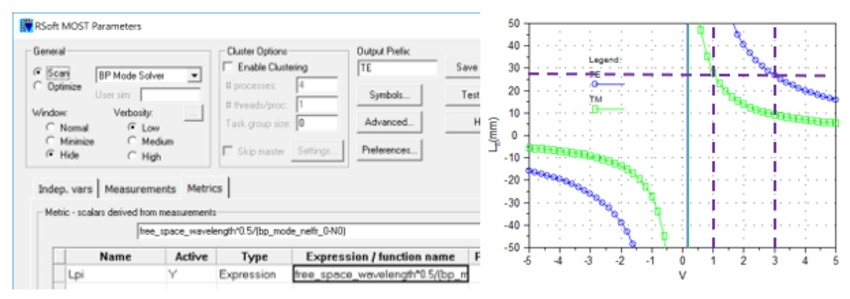Figure 15. MOST Scan of the bias and calculated Lπ.

As predicted, the phase shifter is very polarization dependent because the difference between R13 and R33.

Based on these curves, the half-wave voltage is 1V for a push-pull MZI with 14mm long electrodes, for TM polarization. For TE polarization, however, the half-wave voltage is about 3V. This is consistent with the fact that R13=R33/3.

## Performance of the MZI

With each part designed properly, an MZI can be built and its performance needs to be verified. The whole device can be simulated by BeamPROP, plus Multi-Physics Utility for E-O effect. Shown in Figure 16 are simulation results under 1V bias for both TE and TM waves.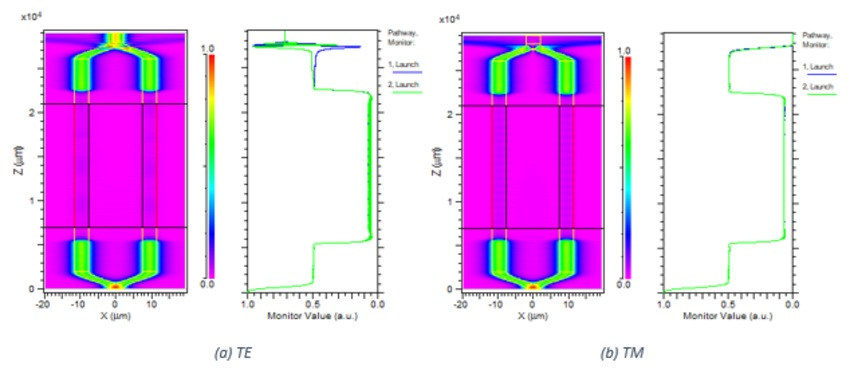Figure 16. BeamPROP simulation results for MZI modulator under 1V bias.

As expected, under 1V bias, the TM wave is in an off-state and TE is not. Because of the long device, one simulation takes about 40 minutes on an 8-core computer. The memory usage is very small (0.05GB).

To test the dynamic performance, we can use MOST to scan over the bias, and the results of both TE and TM waves are shown in Figure 17. As observed, the modulator is very polarization dependent.

In case of 45o linearly polarized input as used in the reference , the input consists of TE and TM waves equally and the performance will be the sum of both, as shown in the same chart.  There are many factors to affect the shape of the combined curve, mostly the difference between R13 and R33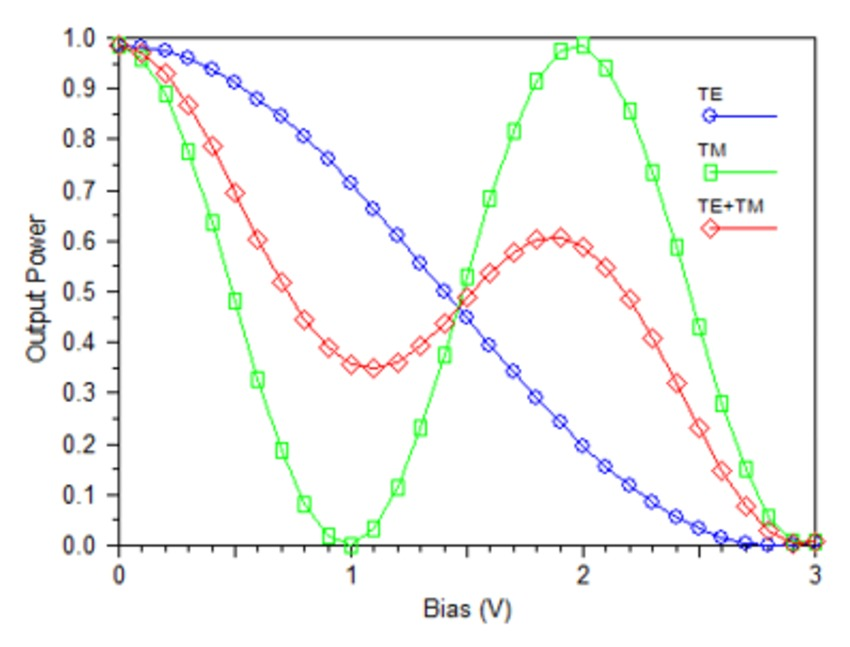Figure 17. Dynamic performance of the MZI modulator.

## Mask Layout

Once the device is optimized, it is ready to export the final design to a mask file, either GDS II or DXF file as shown in Figure 18(a). The output is a multilayer mask and the layer number was assigned for each segment, as shown in the insert additional properties for each segment. The default layer number is 0.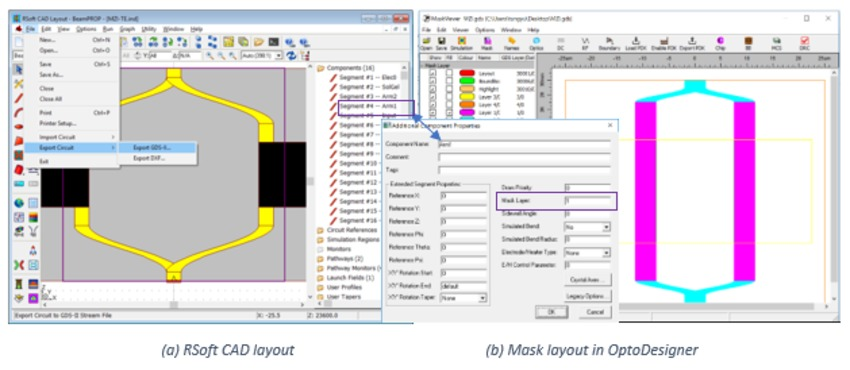Figure 18. Mask layout output.

The exported mask file can be viewed by any mask viewer software. It can be imported into OptoDesigner for further editing, as shown in Figure 18(b).

## Conclusion

It has been demonstrated that RSoft’s BeamPROP is an excellent simulation tool to design sol-gel based E-O modulators. The complex device can be decomposed into a few basic functional blocks and each block can be designed and optimized separately. The overall device performance can also be verified by BeamPROP, though this is a little bit time consuming.

Please be advised that this example is for demonstration purpose only, and is not aimed for direct comparison with the published results, simply because a lot of device parameters are not available in the references. Nevertheless, users can follow the same procedure and work on their own design by using their own design parameters.

## References

 Enami, Y., et al. "Hybrid polymer/sol–gel waveguide modulators with exceptionally large electro–optic coefficients." Nature Photonics 1.3 (2007): 180-185.

 Enami, Y., et al. "Low half-wave voltage and high electro-optic effect in hybrid polymer/sol-gel waveguide modulators." Applied physics letters 89.14 (2006): 143506.

 Enami, Yasufumi. "Fabricating 90 nm Resolution Structures in Sol-Gel Silica Optical Waveguides for Biosensor Applications." Journal of Sensors 2017 (2017).